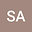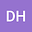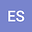Study of the Fragile Points Method for solving two-dimensional linear and nonlinear wave equations on complex and cracked domains
•••Donya Haghighi
Imam Khomeini International University
Author ProfileElyas Shivanian
Imam Khomeini International University
Author Profile## Abstract

In the current research, we present the meshless Fragile Points Method for the two-dimensional linear and nonlinear wave equations on irregular and complex domains. In addition, problems with domains that have cracks, fractures, or any other type of discontinuity have been studied. This method uses a simple Galerkin with the domain divided into subdomains by a Voronoi diagram. Based on selected points in the domain, the method of generalized finite difference is applied for deriving the test and trial functions. These functions are simple, local, and discontinuous polynomials. Discontinuity of test and trial functions may cause FPM method inconsistency. In this study, we apply numerical flux corrections to avoid incompatibilities. Time derivatives of the wave equation are eliminated using finite difference schemes and the theorems of stability and convergence of this semi-discrete scheme are presented. FPM is very suitable for solving equations that have complex, irregular that have fractures, cracks, and rupture domains. Numerical examples of solving a two-dimensional wave equation on domains with complex geometry are presented to illustrate the capabilities of FPM. These examples presented with random and uniform points demonstrate the reliability and robustness of the method at different times. It is also shown how the number of points selected from the domain correlates with the accuracy of the calculations. Also, according to the number of points used, the final time, and the time steps, the method has a good computational speed.